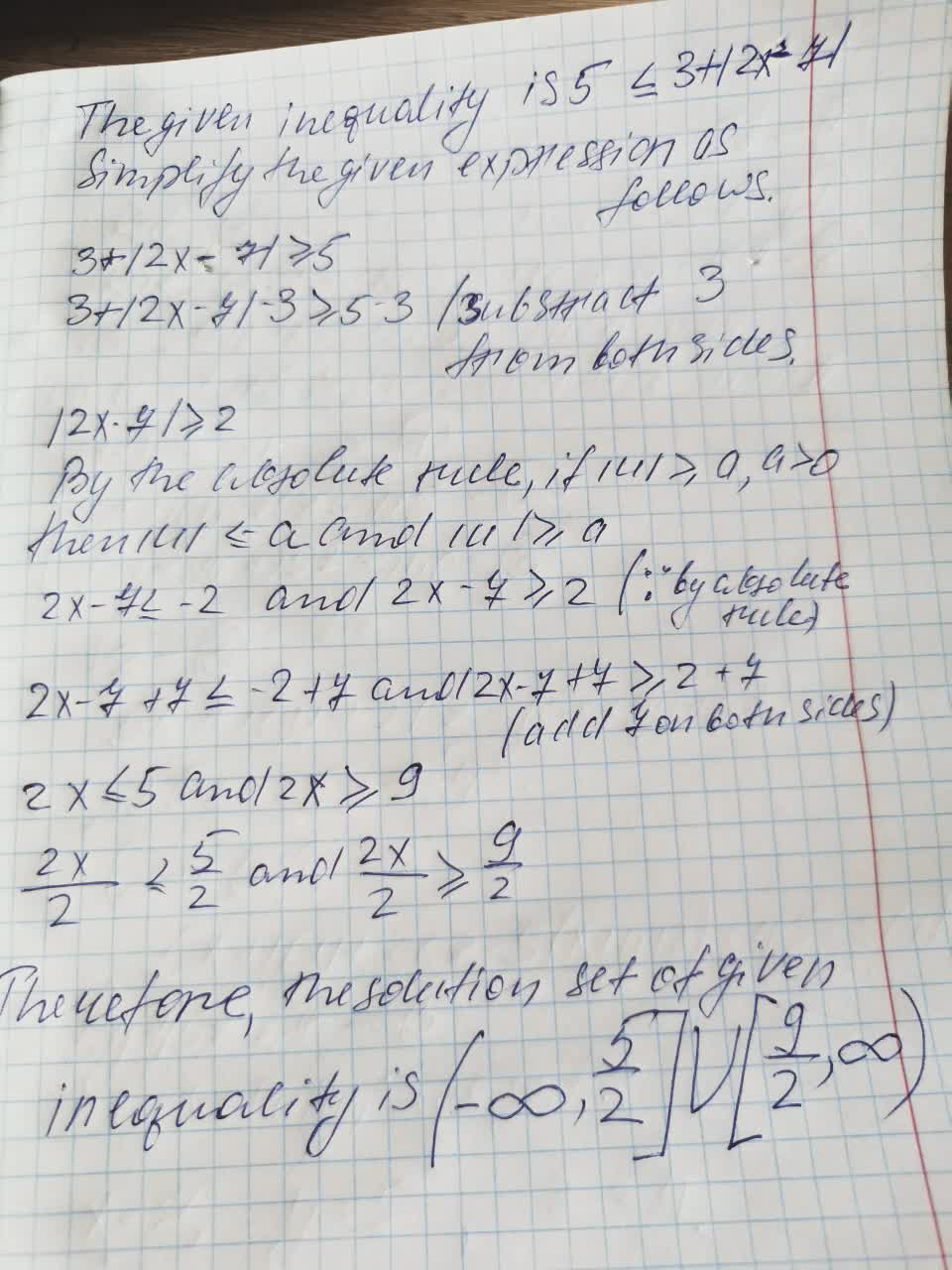# Solve the equations and inequalities. Write the solution sets to the inequalities in interval notation.5 <= 3 + |2x− 7|Dolly Robinson 2021-09-25 Answered

Solve the equations and inequalities. Write the solution sets to the inequalities in interval notation.
$$5 ≤ 3 + |2x− 7|$$

• Questions are typically answered in as fast as 30 minutes

### Plainmath recommends

• Get a detailed answer even on the hardest topics.
• Ask an expert for a step-by-step guidance to learn to do it yourself.Jozlyn
Solving of this inequalitie###### Have a similar question?content_user

Answer is given below (on video)